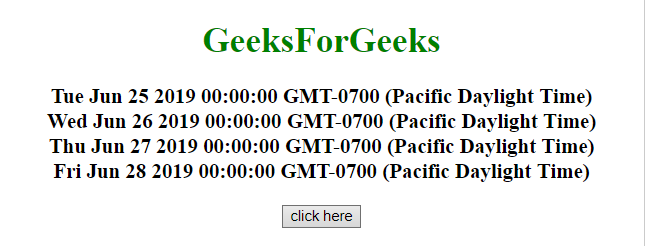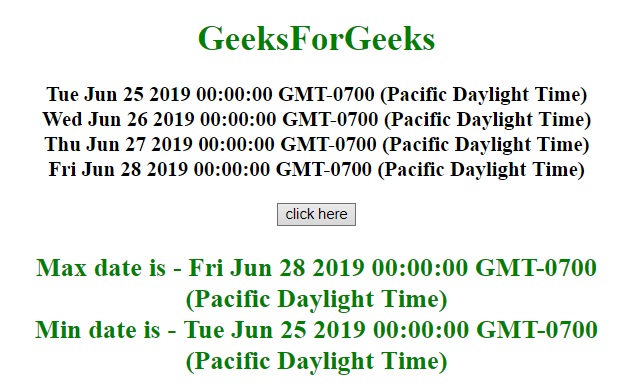# How to select Min/Max dates in an array using JavaScript ?

Given an array of JavaScript date. The task is to get the minimum and maximum date of the array using JavaScript.

Approach 1:

• Get the JavaScript dates in an array.
• Use Math.max.apply() and Math.min.apply() function to get the maximum and minimum dates respectively.

Example: In this example, the maximum and minimum date is determined by the above approach.

 `  ` `<``html``>  ` ` `  `<``head``>  ` `    ``<``title``>  ` `        ``JavaScript | Min/Max of dates in an array. ` `    `` ` `  ` ` `  `<``body` `style` `= ``"text-align:center;"` `id` `= ``"body"``>  ` `     `  `    ``<``h1` `style` `= ``"color:green;"` `>  ` `        ``GeeksForGeeks ` `    `` ` `     `  `    ``<``p` `id` `= ``"GFG_UP"` `style` `= ` `        ``"font-size: 19px; font-weight: bold;"``> ` `    `` ` `     `  `    ``<``button` `onclick` `= ``"GFG_Fun()"``> ` `        ``click here ` `    `` ` `     `  `    ``<``p` `id` `= ``"GFG_DOWN"` `style` `= ` `        ``"color: green; font-size: 24px; font-weight: bold;"``> ` `    `` ` `     `  `    ``<``script``> ` `        ``var el_up = document.getElementById("GFG_UP"); ` `        ``var el_down = document.getElementById("GFG_DOWN"); ` `        ``var dates = []; ` `         `  `        ``dates.push(new Date("2019/06/25")); ` `        ``dates.push(new Date("2019/06/26")); ` `        ``dates.push(new Date("2019/06/27")); ` `        ``dates.push(new Date("2019/06/28")); ` `         `  `        ``el_up.innerHTML = dates + "<``br``>" + dates ` `                ``+ "<``br``>" + dates + "<``br``>" + dates; ` `         `  `        ``function GFG_Fun() { ` `            ``var maximumDate=new Date(Math.max.apply(null, dates)); ` `            ``var minimumDate=new Date(Math.min.apply(null, dates)); ` `             `  `            ``el_down.innerHTML = "Max date is - " + maximumDate ` `                    ``+ "<``br``>Min date is - " + minimumDate; ` `        ``} ` `    ``  ` `  ` ` `  ` `

Output:

• Before clicking on the button:• After clicking on the button:Approach 2:

• Get the JavaScript dates in an array.
• Use reduce() method in an array of dates and define the respective function for the maximum and minimum dates.

Example: In this example, the maximum and minimum date is determined by the above approach.

 `  ` `<``html``>  ` ` `  `<``head``>  ` `    ``<``title``>  ` `        ``JavaScript | Min/Max of dates in an array. ` `    `` ` `  ` ` `  `<``body` `style` `= ``"text-align:center;"` `id` `= ``"body"``>  ` `     `  `    ``<``h1` `style` `= ``"color:green;"` `>  ` `        ``GeeksForGeeks ` `    `` ` `     `  `    ``<``p` `id` `= ``"GFG_UP"` `style` `= ` `        ``"font-size: 19px; font-weight: bold;"``> ` `    `` ` `     `  `    ``<``button` `onclick` `= ``"GFG_Fun()"``> ` `        ``click here ` `    `` ` `     `  `    ``<``p` `id` `= ``"GFG_DOWN"` `style` `= ` `        ``"color: green; font-size: 24px; font-weight: bold;"``> ` `    `` ` `     `  `    ``<``script``> ` `        ``var el_up = document.getElementById("GFG_UP"); ` `        ``var el_down = document.getElementById("GFG_DOWN"); ` `        ``var dates = []; ` `         `  `        ``dates.push(new Date("2019/06/25")); ` `        ``dates.push(new Date("2019/06/26")); ` `        ``dates.push(new Date("2019/06/27")); ` `        ``dates.push(new Date("2019/06/28")); ` `         `  `        ``el_up.innerHTML = dates + "<``br``>" + dates ` `                ``+ "<``br``>" + dates + "<``br``>" + dates; ` `         `  `        ``function GFG_Fun() { ` `            ``var mnDate = dates.reduce(function (a, b) { ` `                ``return a < ``b` `? a : b;  ` `            ``});  ` `             `  `            ``var ``mxDate` `= ``dates``.reduce(function (a, b) { ` `                ``return a > b ? a : b; ` `            ``}); ` `             `  `            ``el_down.innerHTML = "Max date is - " + mxDate  ` `                        ``+ "<``br``>Min date is - " + mnDate; ` `        ``} ` `    ``  ` `  ` ` `  ` `

Output:

• Before clicking on the button:• After clicking on the button:My Personal Notes arrow_drop_upCheck out this Author's contributed articles.

If you like GeeksforGeeks and would like to contribute, you can also write an article using contribute.geeksforgeeks.org or mail your article to contribute@geeksforgeeks.org. See your article appearing on the GeeksforGeeks main page and help other Geeks.

Please Improve this article if you find anything incorrect by clicking on the "Improve Article" button below.